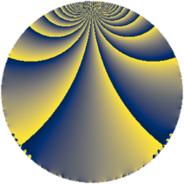# Properties

 Label 126.2.mLevel $126$ Weight $2$ Character orbit 126.m Rep. character $\chi_{126}(41,\cdot)$ Character field $\Q(\zeta_{6})$ Dimension $16$ Newform subspaces $1$ Sturm bound $48$ Trace bound $0$

# Related objects

## Defining parameters

 Level: $$N$$ $$=$$ $$126 = 2 \cdot 3^{2} \cdot 7$$ Weight: $$k$$ $$=$$ $$2$$ Character orbit: $$[\chi]$$ $$=$$ 126.m (of order $$6$$ and degree $$2$$) Character conductor: $$\operatorname{cond}(\chi)$$ $$=$$ $$63$$ Character field: $$\Q(\zeta_{6})$$ Newform subspaces: $$1$$ Sturm bound: $$48$$ Trace bound: $$0$$

## Dimensions

The following table gives the dimensions of various subspaces of $$M_{2}(126, [\chi])$$.

Total New Old
Modular forms 56 16 40
Cusp forms 40 16 24
Eisenstein series 16 0 16

## Trace form

 $$16q + 8q^{4} + 2q^{7} + 12q^{9} + O(q^{10})$$ $$16q + 8q^{4} + 2q^{7} + 12q^{9} - 12q^{11} - 6q^{14} - 8q^{16} - 12q^{18} + 18q^{21} - 48q^{23} - 8q^{25} + 4q^{28} - 12q^{29} - 24q^{30} + 12q^{36} - 8q^{37} - 36q^{39} - 12q^{42} + 4q^{43} + 24q^{46} - 8q^{49} + 60q^{50} + 12q^{51} - 6q^{56} + 48q^{57} - 12q^{58} + 24q^{60} + 24q^{63} - 16q^{64} + 84q^{65} - 28q^{67} + 36q^{74} + 78q^{77} - 24q^{78} - 4q^{79} + 36q^{81} + 18q^{84} - 12q^{85} - 24q^{86} + 24q^{91} - 48q^{92} - 96q^{93} + 12q^{95} - 72q^{99} + O(q^{100})$$

## Decomposition of $$S_{2}^{\mathrm{new}}(126, [\chi])$$ into newform subspaces

Label Dim. $$A$$ Field CM Traces $q$-expansion
$$a_2$$ $$a_3$$ $$a_5$$ $$a_7$$
126.2.m.a $$16$$ $$1.006$$ $$\mathbb{Q}[x]/(x^{16} - \cdots)$$ None $$0$$ $$0$$ $$0$$ $$2$$ $$q+\beta _{10}q^{2}-\beta _{1}q^{3}+\beta _{5}q^{4}+(\beta _{1}-\beta _{6}+\cdots)q^{5}+\cdots$$

## Decomposition of $$S_{2}^{\mathrm{old}}(126, [\chi])$$ into lower level spaces

$$S_{2}^{\mathrm{old}}(126, [\chi]) \cong$$ $$S_{2}^{\mathrm{new}}(63, [\chi])$$$$^{\oplus 2}$$